## Tuesday, November 27, 2007

### Permutations

Hey guys! It's Sharmaine and I'm the scribe for today. It's my first time using slideshare for my scribe post, so I hope it'll help.

Next scribe is... Precious.

## Monday, November 26, 2007

### Combinatorics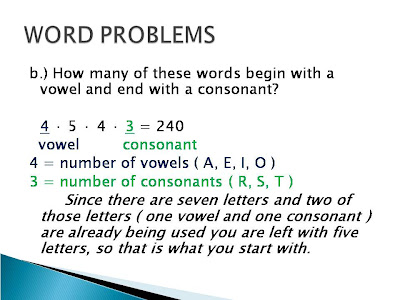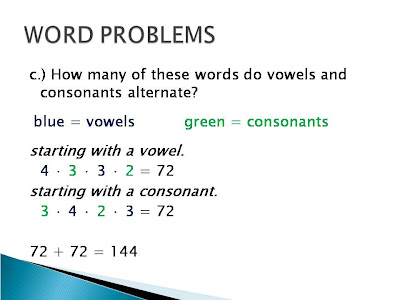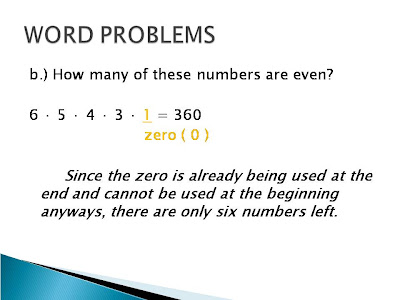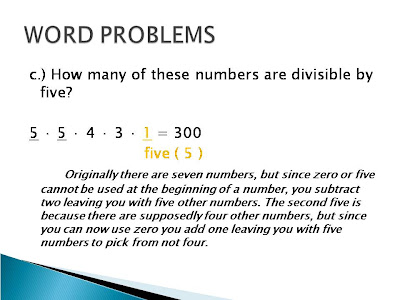Okay, so that was my looong post on combinatories. Sorry for the late post, I was having trouble in how I would publish it. Anyways, I thought I would be able to get away with that really short scribe, but I guess not .. ooh well, it's all good. Oh yeah, before we started class Mr. K showed us this really cool video of some guy figuring out the a rubiks cube; it was craaazy.

HOMEWORK: is to be found one slide down.
and the next scribe is still ..
shane : )

### Today's Slides and Homework: November 26

Here is your homework ...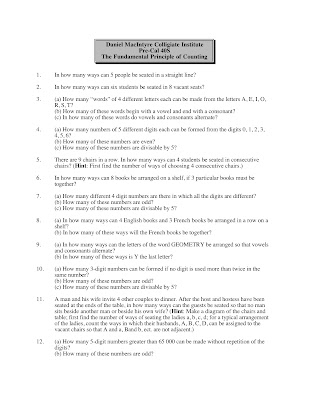Answers are at the bottom of this post.

Here are today's slides ...

Answers to homework questions:

1. 120

2. 20 160

3. (a) 840 (b) 240 (c) 144

4. (a) 2160 (b) 1260 (c) 660

5. 144

6. 4320

7. (a) 4536 (b) 2240 (c) 952

8. (a) 5040 (b) 720

9. (a) 576 (b) 72

10. (a) 891 (b) 445 (c) 179

11. 240

12. (a) 10 416 (b) 5082

## Sunday, November 25, 2007

### TEST DAY

On Thursday, we had a test on Exponents and Logarithms. I hope everyone did well on their test. On Monday, we will continue with our new unit, Combinatories and Functional Notations. That is all :)

the next scribe is .. Sharmaine

## Thursday, November 22, 2007

### BOB

This unit for me was ok, the only challenging concept i found was yesterday doing the review when were were given the log(3)x=y and then your supposed to use the inverse, which is something that I still have no idea why or how you do this. But no complaints I have a rough idea what to do, but this is my muddiest point.

### Blogging on Blogging

I'm a dead man, no doubt. Muddiest point... I'd have to go with everything. If I really wanted to be specific then it'd probably be the half life stuff. This unit was hard, I like the mechanical stuff; this isn't mechanical. It's the darn exponential modeling! I guess everything else I'm alright with, like graphing; piece of the cake. I never know when to use what formula. Like a said: I'm a dead man.

Well then, I'll see you next semester Meesah K. If you feel what I'm saying.

### combinatories and functional notation

Eow this is rosselle. I'm sorry for the late, late, late scribe....anyways...i'll start it with the afternoon class. Mr. K gives us the review for the test and he group us into 6 so here is it:Domain:(2,infinity)
Range:(-infinity, infinity)
y-int: none
x-int: 7/3
aymp:2domain:(-infinity,infinity)
range:(-infinity,1)
aymp:1
y-int:3/4
x-int:2

*ln is like a log. This is change of base law.* We can't expand ln(x-1) and ln(x+3)any further since ln are not distributive!*First I expand the lnx and lny using the product law and by quotient law, I divide lnz.

The class start with Mr. k's social commentaries. He talks about smoking and numbers from the lottery, I don't really get it cause I'm not listening... well anyways we are face with a new lesson and this is what we talked about for the mornin' class...*There are 2 ways for n to be tossed. For every n1 and n2, there are 2 ways that q1 and q2 to be tossed and so that would be 4.
And for every q, there are 2 ways that d1 and d2 to be tossed and so that would be 8.
Now multiply all the numbers :2x2x2=8

*The difference between the Combinatories and Modeling is that combinatories shows how many ways that can be done and arrange items, the following examples show how to use it.*There are 3 ways to wear the P1, P2, P3 then there are P1 x P2 x P3 ways to do three things.*There are 5 people that can be seated in this straight line.
*we used up one number, so we have 4 people to be seated.
*3 people are remaining
*2people remaining
*1person remaining~if ever you want to see the original and clear images just go to this site: www.http://slideshare.net/nocture/inverse-3/

Sorry for the inconvenience in reading my scribe...i know it's blurry....now i can sleep...next scribe is michelle.....thank u thank u...ajah ajah...

### "can't stop B0BB'n ittt" cont`d

After getting off work and getting into some studying because cheyenne is forcing me to, I have just realized... I don't knnow WTH or HOW to use Pe^rt.... ouch like a sucker punch.

## Wednesday, November 21, 2007

### BOB

wellllll in all honesty i think I'm okay for this unit, i understand the concept that log is and exponent and such, graphing is not that much different as i know that say log(base 2)X is the inverse of 2^x.

as for the formulas
i find them to be very easy to use all i need to improve on though is when given little information such as the original amount, half life and the period instead of wasting time on a test trying to solve for rate then using the Pe^rt formula i can just use the model(A2=A1(m)^(t/p)) formula and save some time.

i really think i should start doing far more amount of homework and study for test because i may know this stuff now but say when the exam comes along.. well ill try not to let that happen

well i guess thats it good luck everyone on the test.

### BOB

Hey guys. This is my BOB. Im in the middle of studying right now and im trying to uncover some muddy points. So far, all ive been doing is going through the homework he gave us, once i find areas that i think is difficult, ill tackle them later. I wish you all a good luck on the test!

### BOB

Okay, well I must say that in my opinion, this is the hardest unit so far. Ironically, the concept is so easy, that it is complicated. I think that the most difficult thing about this unit is the word problems. It is hard because I'm not always certain as to which equation I should use. Another thing about this unit that is oddly hard to understand is that "a logarithm is an exponent". Knowing this and actually understanding it can sometimes make a big difference in being able to do the questions, but then again it also the reason as to why this unit is so complicated. That is why I really dislike logarithms. It's like you either get it or you don't. Well I guess that is pretty much it. Everything else that we did is kind of "on and off" for me, sometimes I understand it and sometimes I get questions where I completely draw a blank, but then when it is explained to me I usually understand it again. Good luck on our test guys (:

### BOB

This unit wasn't that hard in the beginning. I think logs and natural logs were not that hard. My muddiest point is the word problems, because I don't know which formula to use. I also had difficulty with graphing the logarithms.
Good luck to everyone on the test tomorrow! :)

### B.O.B.

Hmm, at the beginning of this unit I thought that it was easy. Especially when Mr. K said “A Logarithm is an exponent”. First few questions in this unit was easy but when Mr. K introduce to us the natural logarithm, oh god, it start me to confused but when I remember say the word “A Logarithm is an exponent” over and over again I understand how to solved those problems. But when Mr. K discuss on how to solve the word problems with the three formulas that Mr. K gave to us and how to graph the exponential functions it starts me to confused more. But with the help of my seatmates and the help of the pre-test and the review I barely understand now this unit....

Well, good luck on our exam tomorrow!

### Today's Slides: November 21

Here the morning's slides ...

and from this afternoon ...

### World Population Clock

Comment on what you see here. Try using both audio and text comments. The full video is about one and a half minutes long. You can see the full size version of it here.

### can't stop BOB'n itt

Well, logs don't seem to be too bad, I guess. The flipping around and tricky questions always gets me, and I'll understand things but just freeze up on tests. I just got home from work, and I've been so busy lately that I haven't been able to focus as much as I'd like to, but I just came by the blog to quickly read some things and decided to post my bob. Another thing I have troubles with is (sadly) graphing... I didn't pay attention worth jack in grade 10 and 11, which I wish I did now. So hopefully I can sneak by on that. Well, gotta get a couple of hours of sleep tonight and hopefully be awake for the new unit's intro tomorrow.

## Tuesday, November 20, 2007

### BOB

Logarithms ... hm ... this unit started mellow, in my opinion. It wasn't that hard understanding what a logarithm was in the beginning. But when we started the whole natural log thing and added 'e' and with the problem solving and the graphing and compound interest and three different formulas, i was overwhelmed. I was really glad that we had a quick class discussion yesterday about the feedback from BOB. It really cleared things up for me and I was very relieved to have discussed the questions from the two worksheets that were handed out. Its just really the problem solving that I'm still just getting used to. That's my main weakness, so i guess i better get to work on stretching my brain so i get used to it by thursday... good luck to everyone!

### BOB

Okay, I thought this unit was easy not until Mr. K introduce the natural log. I think my muddiest point are the word problems especially the half-life ones. I get confuse which formula to use. Sometimes I make mistakes with using log or ln in solving for x. The change of base law is pretty easy and the one when you need to solve for x that was an exponent and they have to be in the same base. Good luck everyone!

### BOB

uhm, ello...it's Luis BOB-ing again for the third time. UHm, this unit was really hard,especially the word problems. Most of us are having a hard time to answers those kind of questions. Change of base laws and graphing were quite confusing but after we did a pretty fast recap yesterday and reviewed them again, it kinda give me a hint how to solve them easier when I encounter those kind of stuff on the test. Well, I would say that my muddiest point, again would be WORD PROBLEMS (Huh). Got to go now..i have to burn my eyebrows (study really hard!) still..Goodluck on Thursday guys! Ace the test.

### BOB

This unit was a tough one. At first, when it was being introduced it was simple and easy to follow, but as we got further along into the unit it became very complicated. I have the formulas down, it's just a matter of when I'm supposed to use it and where I plug in the numbers. So, I would have to say my muddiest point was when we started doing word problems; I was so clued out. UGH! aaand I still kind of don't get it. I also had a bit of difficulty with graphing the logarithms, but the review on Monday was great, it helped clear up a lot of things :)

### BOB

For this unit I had trouble with remembering that Logarithms are exponents, not only that but the general idea. I just can't get my head around the whole idea, solving the problems is difficult because you don't know where to start or there's usually a lot of different ways to solve it. When answering word problems I never know what formula to use, it seems like theres 5 and I always get confused with which one is which, constantly compounding, not constantly compounding? I can't recall any other problems I had with this unit, I find graphing to be easy. I try to do my homework at home but like Mr.K said it's extremely better to have study groups because if you don't know the material then you aren't going to just somehow figure it out. I think I need a study group because i don't officially have one. :)

### Scribe Post Logs and Exponents

Mr. K wasn't here today so we had a subsitute by the name of Mr. Roseman. We did our Logs and Exponent pre-test, which was approximately 30 minutes. We then all went into a group of 4-5 and corrected everything and handed our sheets in. Mr. Roseman went over most of the questions with us.

Tomorrow's class we will begin our new unit. Also a reminder, Thursday is our Logs and Exponent test.

Next scribe for tomorrow is .... Luis.

## Monday, November 19, 2007

### BOB Log and Exponents

Sigh* finally this unit is over, at first this is confusing but as it moves along it becomes somewhat easier than what we started. This unit have a lot of confusing things. from exponents to logs to natural logs things. The exponential logs is okay but the formula are hard to firgure out. Its kind of hard to figure out at first which formula to use. I guess the clearest point in this unit for me is the laws like the change of base and other law but the rest were kind of hard for me. The muddiest point is the word problem and also the graphing. Graphing is somewhat hard, I get confused on many things in this unit one of it is graphing. Well I think thats all I can say now since its already late and we have school tomorrow. Good Luck Everyone to the Test!!! I hope all of us wil have good mark on this test. XP

### B-o-B

HELLO!

Bobbin' again... In this unit, Logarithms and exponents, I find it confusing, especially the world problems and graphing. I believe that is my muddiest point. The laws are pretty straight forward. This unit is like trig identities because there's so many ways you can solve a problem. Whatever base you choose. Oh well, that's it for this unit. Good Luck to everyone! Test is on THURSDAY!!!

BYE

### Scribe Post: Logs and Exponents

AM class

Today in our class we did a somewhat review the whole morning. Actually first Mr. K. hand out a new exponetial modeling sheet and most of us, Im just guessing, did not get a good chance to finish the whole sheet that period because majority of the class want to discuss the sheet he hand out last week. Well most of the sheet were explained and I guess everybody kind of understands it. Im not really sure how to explain everything because its mostly straight to the point. Just remember which one is which in using the formula.

The formula we need to memorize are:

P= A (1+ (r/n) ) ^t(n)

A = Pe^rt

A = Ao (model) ^t

Well that's basically what we did in the mornign class. Ooh and we also solve more logarithmic equations.

e^(2t-1) =5

lne^(2t-1) = ln 5

2t-1 = 5

t = (ln 5 + 1) / 2

The other one is this one:

ln (x+1) = 1 + lnx

ln(x+1) - lnx = 1

ln ( (x+1) / x) = 1

e = (x+1) / x

ex = x+1

ex - x = 1

x (e - 1) =1

x = 1 / (e-1)

That's everything we did this morning.

PM class

All we did that afternoon was more review but its all about the things most of us had troubles with.The next were graphing, I cant really explain it because its hard to explain. So far I guess I explained everything. Since we did not really learn something new, we mostly did review today and I hope it somewhat helps to the upsocming test about this unit. Well I guess I covered everything. I have to do other homeworks now.

### B.O.B.

The logarithms and exponent unit felt like FOREVER. I was doing very well in the beginning until we started doing whacky stuff like change of base law and everything after that (thanks to those university stuff). My muddiest point would definitely be the word problems. I actually had a lot of fun in this unit. Well, test is on Thursday. Good luck to everybody.

### BOBBING FOR MATH

I only have one thing to say about Logarithms, EUGH. A big ugly grunt because there's just so many ways you can go about solving just one problem. And because there's so many ways I tend to forget the order of operations and my algebra is off. What I noticed to be difficult for me was solving problems with polynomials as powers because sometimes I'm not sure if I should take the anti log or try to solve it the way it is. I don't like the change of base law either, I wasn't there for that lesson and I tend to get lost now and then. I know how to apply it but I'm still not sure why it works. We discussed it a few times, however it just doesn't seem to stick. The most difficult part is solving word problems. There's different kinds of formulas and I know how to use them it's just sometimes I don't know when to use them and why that one would be a better formula to use as a opposed to a different one.
That's about all the problems I have for the unit Logarithms. Everything else I understood and hope that I remember everything for the test. Wish everyone luck and practice hard.
-SAMUS

### BOB

It is nearly time again for us to write another test. The unit on Logarithms and Exponents has been an interesting one. I think I've got all the different laws memorized. I just need to practice using them more often. My muddiest point for this whole unit would have to be solving problems with "half life" and using the formula A=Ao(1+r/n)^t*n. I also dont really quite understand the new stuff we did today with the decibals. Hopefully I'll do better on this test than I did on all the others.

Ant.. I just had to know that your gonna work out your shoulders tomorrow.

### BOB'in once again

Hey its Ant. Bob'in again. okay the unit of logs. what can i say. This is an enjoyable unit but difficult. A wise man once told me " teachers love to teach logarithms because there are many manipulations you can do with them, students hate logarithms because there are many manipulations you can do with them." That is true. By far i find the consumer math the most difficult, something about it is not the same flavor as Pre-cal. I also find problems involving halflife and solving for the variable T a tad tricky. When you need to find the period and then solve for T. Oh well atleast I know what to study!

No double tommorow!

So Im working Shoulders 4th and Back 5th.

## Sunday, November 18, 2007

### Bobbing on Logarithms

This unit wasn't as hard as the last one, but I'm still having trouble with a lot of things. I guess posting now, at this hour of the night won't really help me to get my problems answered; so this post will just be a reflection on the unit.
I was away for one class where Mr. K taught the change of base law. A fellow classmate explained it to me, but I still didn't get a good idea of it.
What I found extremely useful was when we got into groups again and answered questions on the review sheet. I guess being with the people I was with was a benefit for me since I understood things more clearly and I was actually able to do some of the questions on my own. I was able to do the word problems which I usually have a lot of hard time doing.
A logarithm is an exponent! It's funny how a lot of people, including myself, are still forgetting this very important rule. It's so easily said, but really difficult to remember.

### Bob ing

Okay the unit of logarithms is finally over. I would say that I got maybe 30-40% of the work. All that stuff we did in the beginning i can do pretty easily [or at least i think i can]. The laws, and exponential equations shouldn't be too troublesome. Graphing, and Natural Logarithms [ln] are murky. Not really muddy but its a blur. This is maybe another 30-40% of the unit test? The Word problems which i think is 20-40% of our mark is so so to me. I mean i can remember the formulas for compound interest A=P(1+R/N)^NT and the A=Pe^RT without much trouble. The problem is i don't know where to use which one and even if i did I doubt I would be able to plug in the right numbers for each variable.

Well I hope that my Bob on this unit. If i guesses are correct on how much effects each part is worth than I am in some hot water...

### Log-a-rithms BoB

First of all, every time that Mr. k says that the logarithm is an exponent it makes me really confused, but honestly I beginning to like it, it's hard and complicated but it's fun especially the laws. Because I've been able to manipulate the logs to make it more simple. But I'm a little confused about solving for x and all that stuff. I don't know where to start but I've been working on it. I don't get the exponential modeling, I really need to work on that stuff......oh men... i forgot... I don't like "ln"......wish me luck.....

### Logarithms BOB

Okay, so here's the deelios, I'm not exactly sure if the test is on Monday or not but I was told that it could most likely be on Monday so I will now write this blogging on blogging blog. Logarithms seemed really short for some reason but that's because many things occurred throughout this unit and what I mean by that is I had extra curricular activities going on during the times we had classes and I apologize for that. One thing to really remember though is that logarithms are exponents and I myself forget that time to time and then you end up getting a brain malfunction and you wouldn't be able to answer certain questions if that doesn't sink in your head, but I mean it is kinda hard to remember. Aside from that, if the half life questions are on the test then that's what people should watch out for because if they don't get the hang of it, then it gets tricky, because there are a few equatioPublish Postns you need to understand and use, and it applies the log function and the ln function so it does get tricky. Anyway good luck to all and may you all pass with flying colors in the next test.

### Log and Exponents BoB

B-B-Bobbing.
Well this unit wasn't that difficult in my opinion, but maybe that's just because I'm trying to concentrate more. I think the most difficult concept to grasp at first was that a logarithm is an exponent. Just now I was doing a problem: 27^log[base3]9 (How am I suppose to type that?). I remember we had a similar question on a quiz, which I got wrong. I get it now though because a logarithm is an exponent therefore log[base3]9 is equal to 2. So solving the question is easy because you just take 27^2. It's such a simple concept, but so difficult to grasp! I suppose that's my "breakthrough" moment. Hopefully I can always keep this is mind. "A logarithm is an exponent."
I've been studying diligently this weekend (for the most part) and doing the problems from the textbook. It isn't that difficult especially when they show you how to do it at the back when you get stumped. I've attempted these exercises before, but now I'm trying them all over again so hopefully this proves to be beneficial.
I understand how to do the questions when I have my notes in front of me for quick reference, but I am ultimately worried that I will forget when and where to apply the laws and rules. There's that math anxiety.
Well, the one thing I am confused about at the moment is when to use the "A=Pe^rt" method or the "A=P(1+r/n)^tn" method when problem solving. I think I understand the model stuff though. I'm going to have to work on those problem solving questions.
I've noticed from that my percentage mark on tests is slightly higher on each passing test than the one previous to it. So hopefully by studying diligently, I might pass this test. A little sad, but I'm hoping I do well.
Well, this has been my, b-b-bob. Good luck on the test everyone.
Good day.

### Logs and Exponents BOB

Logarithms and Exponents... is the most difficult unit in all of math history! When we can make both sides have the same base, how does that make it equal? When we were doing the base 'e' stuff... when do I use 'ln' instead of 'log'? Then we got to the word problems... Holeh camolly... We got so many different formulas. I don't even know when to use which formula. On the sheet he gave us on Friday, number two, I just did not get that stuff. Like writing an equation with the data given to you... I just do not know.

### November 16, 2007 : Scribe

i profusely apologize for the long overdue scribe. on friday, sadly, the smartboard was not working =( ... i then realized how much trouble i have without the class discussions. Mr. K gave us a worksheet to work on in groups. Honestly, I was stumped. I'm really having trouble with applying the different formulas in the word problems. There was a heated discussion on whether one formula (a=Pe^rt) can only be used when dealing with money. I agreed on that in the beginning because Sergio made a good point about not being about to "compound" a year/s ( i say that because when i hear/see the word compound, i usually think of interest and principals and that usually comes up when dealing with money and not time). But in question number 2 letter b i disagreed because i actually used the the a=Pe^rt formula and it worked for me. I'm sorry I can't really say anything else because I am still trying to figure it out. I'm truly waiting for Monday's class so I can get some clarity! Hope everyone had a nice weekend and don't forget to BOB because the test is soon. For the next scribe, I choose Remyshire.

## Saturday, November 17, 2007

### Logs and Exponents BOB

It's the end of the third unit and it's time for blogging on blogging. I would have to say that this unit was probably the most difficult because even though Mr.K would say "a logarithm is an exponent", the concept is easy to understand, it's applying it that is confusing. Problems were difficult at first to solve because we didn't learn the rules in class yet. It got easier when Mr.K would give us problems all the time and it really helped with my understanding of this unit. Like the other units, I liked this one since there are so many things you can do with it, such as the exponential modeling. I guess what would make the unit test hard is not knowing when to use the exponent laws or how to use them.

## Thursday, November 15, 2007

### Exponential Modeling

Hey guys, it’s Jordan scribing for today. The class started off with exponential modeling and stories about wolves and deer.
f(x)= ab^x is the basic function
How we model real life situations depends on what kind, or how much information we are given.

When you have:
Limited Information
A= A_0 (model)^t

Lots of Information
A= A_0 〖(m)〗^(t/p)
A = amount of the “substance” at the end of the time period.
A_0 = original amount of the “substance” at the beginning of the time period
Model = is our model for growth or decay of the “substance”, it is usually an exponential expression in base 10 or e although any base can be use.
t = is the amount of time that has passed.
m = is the multiplication factor
p = is the period; the amount of time required to multiply by “m” once

Okay, so having that information we can now solve the following problems.

The first problem has Limited Information.
The population of the earth was 5.3 billion in 1990. In 2000 it was 6.1 billion.
Model the population growth using an exponential function.
What was the population in 2008?

Before solving this problem, Mr. K showed us a really cool stuff in the internet. It is a world population clock. Here’s the link to the site World Population Growth .

Anyways here’s how you solve it:
There are two ways we solved this one with base 10 and the other with base e although any base can be use.
A= A_0 (model)^t
6.1=5.3(model)^10
6.1/5.3= 〖model〗^10

Base 10
log(6.1/5.3)⁡〖= log⁡〖(〖model)〗^10 〗 〗
(1/10) log⁡(6.1/5.3)=log⁡〖(model)〗
0.0061= log⁡(model)
〖10〗^0.0061=model
P=5.3(〖10〗^(0.0061t) )

Base e
ln⁡(6.1/5.3)=ln⁡〖(model)^10 〗
(1/10) ln⁡〖(6.1/5.3)=ln⁡(model) 〗
0.0141= ln⁡(model)
e^0.00141=model
P=5.3(e^(0.0141t) )

∴ 〖10〗^(0.0061)= e^(0.00141)=1.0142
e^x
*Solving this kind of problem with base e is preferable because the exponent in base e, when you rewrite it in percent, tells you the percent rate of growth or decay per time.

t=0 in 1990
t=18 in 2008

P=5.3(〖10〗^(0.0061t) )
P=5.3(〖10〗^((0.0061)(18) )
P=6.8261
P=5.3(e^(0.0141t) )
P=5.3(e^((0.0141)(18)) )
P=6.8261

Okay, so moving on to the next problem which we are given Lots of Information.
A colony of bacteria doubles every 6 days. If there were 3000 bacteria to begin with how many bacteria will there be in 15 days?
A= A_0 〖(m)〗^(t/p)
A=〖3000(2)〗^((15/6) )
A=16970.5628
A ≈16971
Okay so that it is for our morning class.

Now for our afternoon class, we watched a short movie clip about star trek and tribbles. Here’s the link for the clip mailto:http://youtube.com/watch?v=lZvmxmVVdk8.
Here is another example of exponential modeling given with Lots of Information.
The mass in (grams) of radioactive material in a sample is given by:
M(t)= 100e^(-0.0017t)
where t is measured in years.
Find the half-life of this radioactive substance.
Create a model using the half-life found in (a). How much of a 10 gram sample of the material will remain after 40 years?

M(t)= 100e^(-0.0017t)
50= 100e^(-0.0017t)
1/2= e^(-0.0017t)
ln⁡〖(1/2)=ln⁡〖e^(-0.0017t) 〗 〗
ln⁡(1/2)= -0.0017t
(1/(-0.0017)) ln⁡(1/2)=t
t=407.7336 years
The half-life of this radioactive substance is 407.7336 years approximately.
ALWAYS REMEMBER THAT A LOGARITHM IS AN EXPONENT!
A= A_0 〖(m)〗^(t/p)
A=10(1/2)^((t/407.7336) )
A=10(1/2)^((40/407.7336) )
A=9.3426 grams
OR
A= e^(-0.0017t)
A= e^((-0.0017)(40))
A=9.3426 grams

*Most of the class didn`t know the story about the life of ants and wasps. So Mr. K had to tell how they grow exponentially.*

So that’s it for Exponential Modeling. Let’s now go back to the Consumer Math stuff.
We’re given this problem to solve:
A \$5000 investment earns interest at the annual rate of 8.4% compounded monthly.
What is the investment worth after one year?
What is it worth after 10 years?
How much interest is earned in 10 years?

We use this formula in compound interest.

A = The new Amount
P = Principal/original amount
R = The rate of which it will accumulate
N = Number of times per time amount given (rate will be divided by this value)
T = Amount of Time that it has been accumulating for (yearly, seconds, months, whatever it may be)
What is the investment worth after one year?
A=5000(1+0.084/12)^12
A=\$5436.55
What is it worth after 10 years?
A=5000(1+0.084/12)^(12*10)
A=5000(1+0.084/12)^120
A=\$11547.99

How much interest is earned in 10 years?
I_T=A-P
I_T= \$11547.99-\$5436.55
I_T=\$6111.44
Okay so that’s pretty much all we did this day. I hope my scribe post helped you!
Ciao

NEXT SCRIBE is
CHRYCEL

the better version ( i think) of my post is

here....

## Wednesday, November 14, 2007

### Logarithms and Exponents

Hey guys, it's Luis posting. Today we start off talking about narrowing our votes from 16 down to 7, to belong some of our scribe posts into the hall of fame...and we decided that 2 points for the outsiders, mentors or teachers. And then Mr. K had given us some problems to sketch their graphs... here are they are:We should be able to picture what y= e^x looks like.. and WE can easily figured out two points (0,1) and (1,e), e which is a little bit of less than three. In y=e^x-2, we just have to shift our graph two units down. our asymptote is y=-2, our graph should not cross over that line.
In number two problem, draw first an imaginary line of y=e^x, then -e = we just have to flip it from up to down but over the x-axis because e is negative, -x= we just flip it from right to left. and our asymptote is y=0.

In the last problem, we have to start ignoring the absolute value, then graph the e^x-1...then the last thing we should do is to figure out what is the absolute value. (that was easy! huh.) Our asymptote this time is not equal to -1, it should be 1 because of the absolute value.

Anyways, our prof then discussed the properties of Exponential and Natural Log of Functions by comparing what y=e^x and y=ln(x) looks like...visit our number one graphing online tool,

Whapak!, yes! we're on the right track, y=ln(x) is the inverse function of y=e^x. Haha. I never thought of that, do you? Anyways, we then easily understand what is the difference of their domain and range, we just have to switch them.

One of our classmate, answered this question easily...BUT...but Mr. K. is looking for the two points, (1,0) and (e,1) which (-1,0) and (-e,1). And our asymptote is x=0 because it is ln. Her answer was brilliant though.
Then Mr. K had given us a problem, which terrified us (not really, hehe)...but confused us a lot and asked him lots of questions...
But then explained us that the exponent on the base e that gives us e^x... will give us x. We should really recognize that ln of e^x is equal to x. Then apply the power law...Even though we used the common logarithm (right side) their answers are both the same.

Before our period ends, Mr. K. had introduced us to our new topic, Exponential Modeling. Bare with me guys, but I didn't get most of it...but I'm pretty sure he will talk about this again tomorrow. Ask him lots of questions...OK? And don't forget guys, Logarithms are Exponents.

That's all about we learn this morning...The next Scribe is Jordan.

### A little help... would help.

Hey, does anyone know how to do question 11 on Exercise 25? I didn't post this in the chat box 'cause I doubted anyone would see it there. &anyone know how to even start doin' Ex. 26?? Any comments/replies would be appreciated (though, I kinda doubt that's goin' to happen).

## Tuesday, November 13, 2007

### Scribe Post - Logs and Exponents

Today we start off with some review on logs and exponents: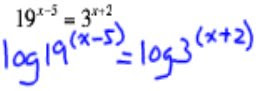looking at 19^(x-5)=3^(x+2) we know that to get an exact answer we must get the log of both sides..

which then becomes: Log19^(x-5)=Log3^(x+2)

REMEMBER: That Log is an exponent

so when an exponent is increasing to another exponent we can multiply them:which we can then expand and move x to one side which becomes: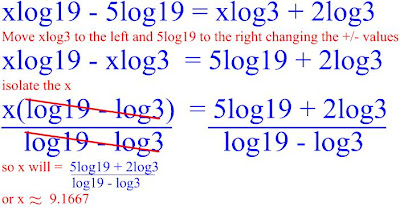now something a little new....

well first what is compound interest?

compound interest is growth from the original amount by a certain rate that will continue to grow exponentially from the last amount

we were given a formula that can help us calculate this value:A = The new Amount
P = Principal/original amount
R = The rate of which it will accumulate
N = Number of times per time amount given (rate will be divided by this value)
T = Amount of Time that it has been accumulating for (yearly, seconds, months, whatever it may be)

knowing that we can plug in what ever information is given to find what we need to know: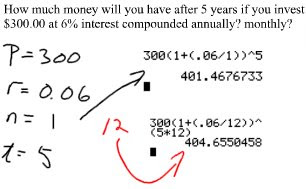we see that that question sayes "How much money will you have after 5 years if you invested \$300" "5 years" becomes our T value and "\$300" becomes our P value

then its goes to say"at 6% interest compounded annually? monthly?"

T=5

P=300
well r=0.06 (6%)

n=1 annually means yearly

n also will = 12 (monthly)

which means you have use this formula twice to get a monthly and yearly amount

now when this interest is compounded continuously we would use a different formula but will look very much the same: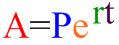and so in this next question we can see how we would use it.first question is pretty straight forward, much like the last one we did only difference is that it is compounded continuously. Sooo p=300 r=0.06 t=5

the second question ask if you made if you have invested \$300(P=300) at 6%(r=0.06) how long will it take to get double your money (A=600). so it wants to no T time it will take to get \$600
600 and 300 will reduce to 2 and 1:
2 = e^0.06t
if we take the ln (log e log base e) of both sides then divide by 0.06 we can isolate t
ln2=lne^0.06t

ln2 =0.06t
0.06 0.06

ln2 = t
0.06

that's about all we learn in the morning in the afternoon we had a short log quiz and then we did exercise the next for the rest of the class time

The Next Scribe Isssssssssssss..................... not lina as i said before, but instead will

be luis voluntarily.

sorry for the mix up i was in a bit of a rush

## Monday, November 12, 2007

### Logarithms and Exponents

Hey everyone, this is JessiccaI as scribe for today's class. At the beginning of class we started off with two questions.

The first question:Using the Product law, you can multiply the powers of the logs together.In the second line, you notice that the equation is in exponential form. You put it into exponential form since you have the base and the exponent already. Once both sides have been multiplied out, you make everything equal to zero. Since it doesn't factor well, you can pull out the Quadratic Formula to solve what x is:You can always check if your answers are correct if you plug them correctly into you calculator or if you approximate the values of x. Note: if you plug it into you calculator make sure the you've made the whole equation into base 10 (the common log).

The second question we got was: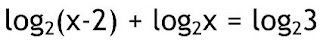This almost the exact same thing as the one above. The only exception being that both sides of the equations have logs. The answer for this question:The "antilog" on the third line undoes the log on both sides of the equation, so you're left with a quadratic. The -1 was rejected because when plugged in to the original equation Log(base)2 (of) (x-2), it becomes a negative. No number can equal a negative no matter how many times the number gets multiplied by itself.

After finishing these questions we then looked at the Properties of Exponential Functions and the Properties of Logarithmic Functions. Refer to November 12, 2007 slides to see the graphs and properties of the graphs. Remember if you want to see a log graph on the calculator or calculate values, make everything to base 10 or, in other words, use the Change of Base Law we learned to do on Friday.

The next scribe is
JoeS.